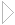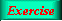Lecture 2 - Page 46 : 46
 Functional Programming in SchemeExpressions, Types, and Functions * Lisp and Scheme Lisp Scheme * Expressions and values Expressions, values, and types Examples of expressions and their values Evaluation of parenthesized expressions Arithmetic expressions Equality in Scheme The read-eval-print loop * Types Types Type checking Static type checking An example of type checking Types in functional programming languages * Lists Proper lists Symbolic expressions and improper lists Practical list construction List functions Association lists Property lists Tables as lists of rows Programs represented as lists * Other Data Types Other simple types Vectors Strings * Definitions Definitions * Functions The function concept Lambda calculus Functions in Scheme Function objects Functions as first class values Anonymous functions Lambda expressions in Scheme Optional parameters of Scheme functions (1) Optional parameters of Scheme functions (2) Closures Function definition in Scheme Simple web-related functions (1) Simple web-related functions (2) Function exercises
 Function exercisesColors in HTMLLetter case conversion